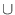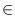Next: Minimum Spanning Trees ( Up: Graph Algorithms Previous: General Weighted Matchings (   Contents   Index

# Stable Matching ( stable_matching )

We are given a bipartite graph G = (AB, E) in which the edges incident to every vertex are linearly ordered. The order expresses preferences. A matching M in G is stable if there is no pair (a, b)EM such that (1) a is unmatched or prefers b over its partner in M and (2) b is unmatched or prefers a over its partner in M. In such a situation a has the intention to switch to b and b has the intention to switch to a, i.e., the pairing is unstable.
We provide a function to compute a correct input graph from the preference data, a function that computes the stable matching when the graph is given and a function that checks whether a given matching is stable.

 void StableMatching(const graph& G, const list& A, const list& B, list& M) The function takes a bipartite graph G with sides A and B and computes a maximal stable matching M which is A-optimal. The graph is assumed to be bidirected, i.e, for each (a, b)E we also have (b, a)E. It is assumed that adjacency lists record the preferences of the vertices. The running time is O(n + m). Precondition The graph G is bidirected and a map. Sets A and B only contain nodes of graph G. In addition they are disjoint from each other. bool CheckStableMatching(const graph& G, const list& A, const list& B, const list& M) returns true if M is a stable matching in G. The running time is O(n + m). Precondition A and B only contain nodes from G. The graph G is bipartite with respect to lists A and B. void CreateInputGraph(graph& G, list& A, list& B, node_map& nodes_a, node_map& nodes_b, const list& InputA, const list& InputB, const map >& preferencesA, const map >& preferencesB) The function takes a list of objects InputA and a list of objects InputB. The objects are represented bei integer numbers, multiple occurences of the same number in the same list are ignored. The maps preferencesA and preferencesB give for each object i the list of partner candidates with respect to a matching. The lists are decreasingly ordered according to the preferences. The function computes the input data G, A and B for calling the function StableMatching(constgraph&,...). The maps nodesa and nodesb provide the objects in A and B corresponding to the nodes in the graph. Precondition The entries in the lists in the preference maps only contain elements from InputB resp. InputA. There are no multiple occurences of an element in the same such list.Next: Minimum Spanning Trees ( Up: Graph Algorithms Previous: General Weighted Matchings (   Contents   Index